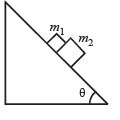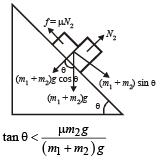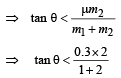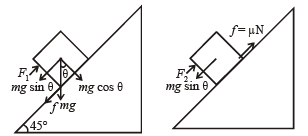Courses

# Matrix-Match & Integer Answer Type Questions: Laws of Motion | JEE Advanced Notes | EduRev

## JEE : Matrix-Match & Integer Answer Type Questions: Laws of Motion | JEE Advanced Notes | EduRev

The document Matrix-Match & Integer Answer Type Questions: Laws of Motion | JEE Advanced Notes | EduRev is a part of the JEE Course Physics 35 Years JEE Main & Advanced Past year Papers.
All you need of JEE at this link: JEE

Match the Following

DIRECTIONS (Q. No. 1) : Following question has matching lists. The codes for the lists have choices (a), (b), (c) and (d) out of which ONLY ONE is correct.

Q.1. A block of mass m1 = 1 kg another mass m2 = 2 kg, are placed together (see figure) on an inclined plane with angle of inclination θ. Various values of θ are given in List-I. The coefficient of friction between the block m1 and plane is always zero. The coefficient of static and dynamic friction between the block m2 and the plane are equal to μ = 0.3. In List-II expressions for the friction on block m2 are given. Match the correct expression of the friction in List-II with the angles given in List-I, and choose the correct option. The acceleration due to gravity is denoted by g.[Useful information: tan (5.5°) ≈ 0.1; tan (11.5°) ≈ 0.2; tan (16.5°) ≈ 0.3]

 List-I List-II P. θ = 5° m2g sinθ Q. θ = 10° (m1 + m2)g sinθ R. θ = 15° μm2g cosθ S. θ = 20° μ(m1 + m2)g cosθ

Code:

(a) P-1, Q-1, R-1, S-3
(b) P-2, Q-2, R-2, S-3
(c) P-2, Q-2, R-2, S-4
(d) P-2, Q-2, R-3, S-3

Ans. (d)

Solution. If (m1 + m2) sin θ < μN2 the bodies will be at rest i.e., (m1 + m2)g sin θ < μm2 g cos θ⇒ tan θ < 0.2 i.e.,

If the angle θ < 11.5° the frictional force is less than

µN2  = µm2g = 0.3 × 2 × g = 0.6 g

and is equal to (m1 + m2)g sin θ

At θ = 11.5° the bodies are on the verge of moving, f = 0.6 g

At θ > 11.5° the bodies start moving and f = 0.6 g

The above relationship is true for (d).

Integer Value Correct Type

Q.1. A block is moving on an inclined plane making an angle 45° with the horizontal and the coefficient of friction is μ. The force required to just push it up the inclined plane is 3 times the force required to just prevent it from sliding down. If we define N = 10 μ, then N is                    (2011)

Ans. 5

Solution.The pushing force F1 = mg sinθ + f

∴ F1 = mg sin θ + μ mg cos θ  = mg (sin θ + μ cos θ)

The force required to just prevent it from sliding down

F2 = mg sin θ – µN = mg (sin θ – μ cos θ)

Given ,   F1 = 3F2

∴ sin θ + μcos θ = 3(sin θ – μ cos θ)
∴ 1 + μ = 3(1 – μ)  [∴ sin θ = cos θ]
∴ 4μ = 2
∴ μ = 0.5
∴ N = 10 μ = 5

Offer running on EduRev: Apply code STAYHOME200 to get INR 200 off on our premium plan EduRev Infinity!

88 docs|49 tests

,

,

,

,

,

,

,

,

,

,

,

,

,

,

,

,

,

,

,

,

,

;